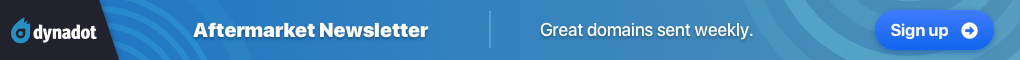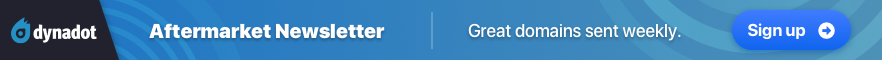# domaindubaicheapesthotels.comStatus
Not open for further replies.

1. ### Quick Reply

•••
The views expressed on this page by users and staff are their own, not those of NamePros.

Up
^^^^^
^^^^
^^^
^^
^
^^
^^^
^^^^
^^^^^
UP

•••

Up
^^^^^
^^^^
^^^
^^
^
^^
^^^
^^^^
^^^^^
UP

•••

Up
^^^^^
^^^^
^^^
^^
^
^^
^^^
^^^^
^^^^^
UP

•••

Up
^^^^^
^^^^
^^^
^^
^
^^
^^^
^^^^
^^^^^
UP

•••

Up
^^^^^
^^^^
^^^
^^
^
^^
^^^
^^^^
^^^^^
UP

•••

Up
^^^^^
^^^^
^^^
^^
^
^^
^^^
^^^^
^^^^^
UP

1. ### Quick Reply

•••
Status
Not open for further replies.